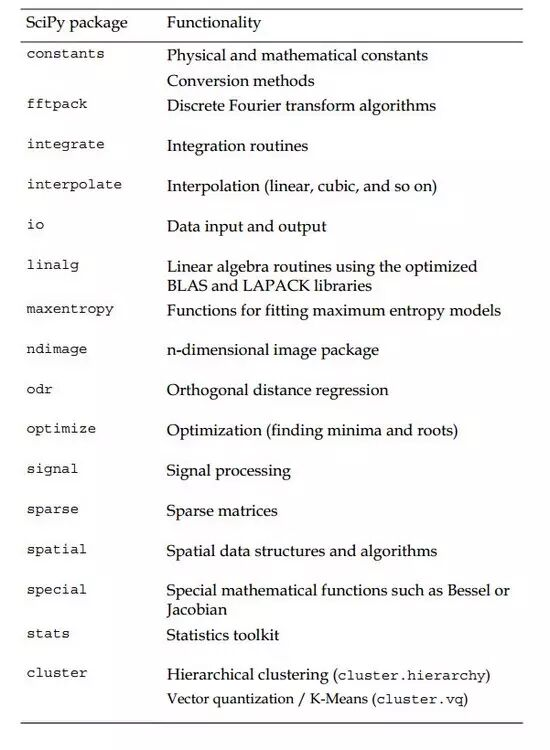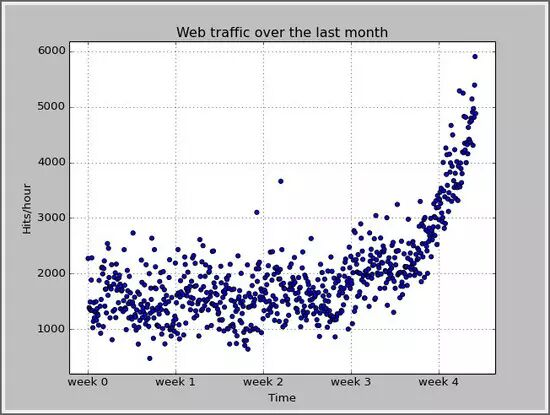# 用Python进行机器学习实例

### 概要

Python是一个高度优化的解释性语言，在处理数值繁重的算法方面要比C等语言慢很多，那为什么依然有很多科学家和公司在计算密集的领域将赌注下在Python上呢？因为Python可以很容易地将数值计算任务分配给C或Fortran这些底层扩展。其中NumPy和SciPy就是其中代表。NumPy提供了很多有效的数据结构，比如array，而SciPy提供了很多算法来处理这些arrays。无论是矩阵操作、线性代数、最优化问题、聚类，甚至快速傅里叶变换，该工具箱都可以满足需求。### 读入数据操作

```import scipy as sp
data = sp.genfromtxt('web_traffic.tsv', delimiter='\t')```

### 预处理和清洗数据

```hours = data[:,0]
hits = data[:,1]
sp.sum(sp.isnan(hits))```

```#cleaning the data
hours = hours[~sp.isnan(hits)]
hits = hits[~sp.isnan(hits)]```

```import matplotlib.pyplot as plt
plt.scatter(hours,hits)
plt.title("Web traffic over the last month")
plt.xlabel("Time")
plt.ylabel("Hits/hour")
plt.xticks([w*7*24 for w in range(10)],
['week %i'%w for w in range(10)])
plt.autoscale(tight=True)
plt.grid()
plt.show()```### 选择合适的学习算法

```def error(f, x, y):
return sp.sum((f(x)-y)**2)```

`fp1, residuals, rank, sv, rcond = sp.polyfit(hours, hits, 1, full=True)`

fp1是polyfit函数返回模型参数，对于直线来说，它是直线的斜率和截距。

```#fit straight line model
fp1, residuals, rank, sv, rcond = sp.polyfit(hours, hits, 1, full=True)
fStraight = sp.poly1d(fp1)
#draw fitting straight line
fx = sp.linspace(0,hours[-1], 1000) # generate X-values for plotting
plt.plot(fx, fStraight(fx), linewidth=4)
plt.legend(["d=%i" % fStraight.order], loc="upper left")```

```Error of straight line: 317389767.34
Error of Curve2 line: 179983507.878
Error of Curve3 line: 139350144.032
Error of Curve10 line: 121942326.364
Error of Curve50 line: 109504587.153```

### 回看数据

```inflection = 3.5*7*24 #the time of week3.5 is an inflection
time1 = hours[:inflection]
value1 = hits[:inflection]
time2 = hours[inflection:]
value2 = hits[inflection:]
fStraight1p = sp.polyfit(time1,value1,1)
fStraight1 = sp.poly1d(fStraight1p)
fStraight2p = sp.polyfit(time2,value2,1)
fStraight2 = sp.poly1d(fStraight2p)```

### 总结

1、要训练一个学习器，必须理解和提炼数据，将注意力从算法转移到数据上

2、学习如何进行机器学习实验，不要混淆训练和测试数据

PythonTab微信公众号:Python技术交流互助群 ( 请勿加多个群 ):